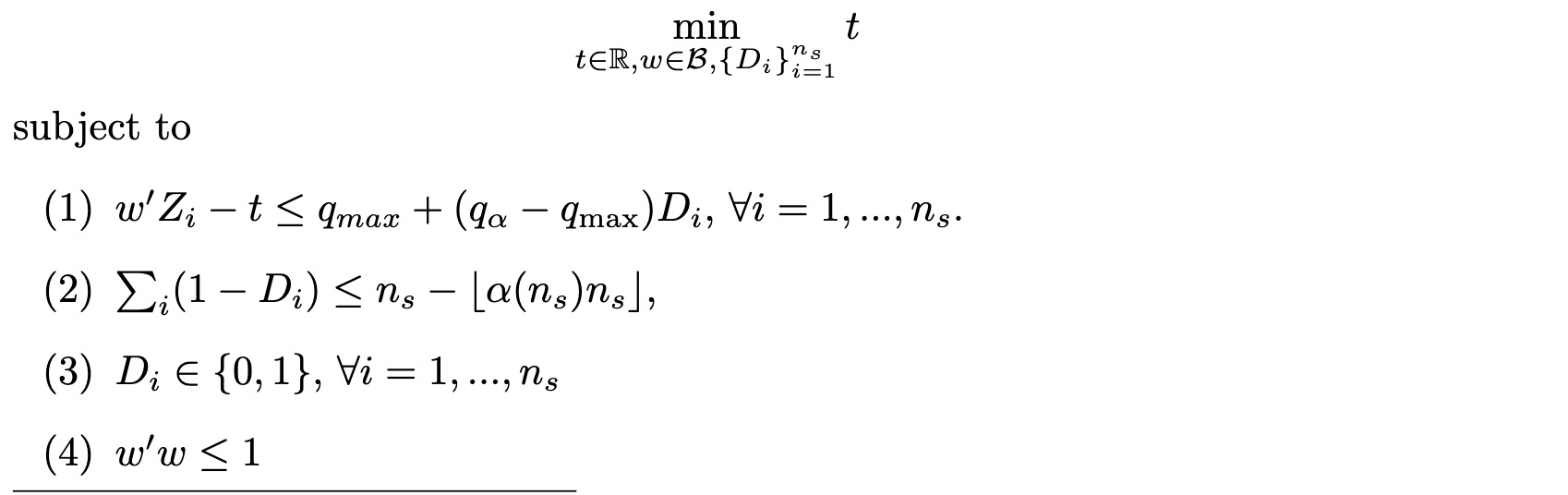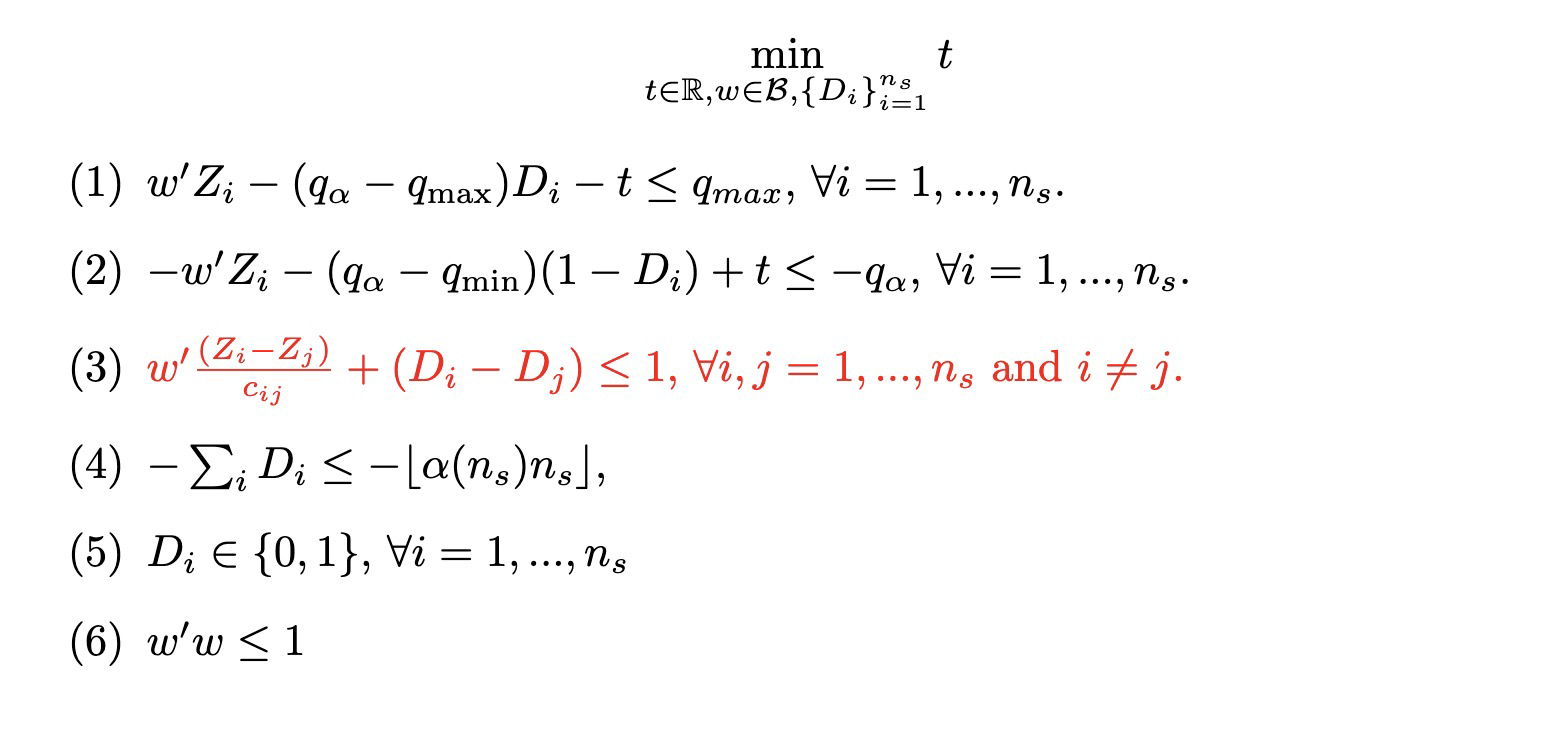•Gurobi Staff

Hi,

The behavior you see makes sense. The reason is the constraint

I have one quadratic constraint that restricts the continuous variables on a unit ball.

which most likely introduces a lot of symmetry into the model. The best way to deal with the symmetry is to introduce an ordering of variables, e.g., $$x_1 \leq x_2 \leq x_3 \leq ...$$.

Out of curiosity, could you please share the model? Maybe there is something we could improve on. Note that uploading files in the Community Forum is not possible but we discuss an alternative in Posting to the Community Forum.

Best regards,
Jaromił

•Hi Jaromił,

Thank you for your prompt response!

1. I want to check how the BestBound is generated. Since my problem is a minimization problem, the Bestbound is the minimum (not the maximum) of all relaxations at the leaf nodes, right? Does it mean that, if the Bestbound is not improving, there are lots of leaf nodes that need to be explored?

2. Regarding the ordering constraints, there is no guarantee that a particular ordering is optimal for my problem. Trying out all (#continuous variable)! seems impossible.

3. Here is the link to the replication files

'Trial_0919.m' will replicate the results I posted above.

The file 'CR_draw.m', 'D_cr.m', and 'gen_diag_matrix_cr.m' are auxiliary files that generate inputs to the main file.

'Trial_0911.m' solves the same problem as the one in 'Trial_0919.m', but with fewer constraints. Unfortunately, Trial_0911.m works better than Trial_0919.m based on my experiments. Trial_0911.m converges in one hour on a computer node with 48 cores. I have not tried Trial_0919.m, but on my computer Trial_0919.m performs worse.

Finally, let me briefly mention the setup of my problem. This is a Value-at-Risk problem, where I want to minimize the quantile of a linear combination of random variables with weights that square-sum to 1.

Decision Variable:

1. w (continuous): a n-dimensional vector restricted on a unit ball;

2. D_i, i=1,...,n_s: binary variable

3. t (continuous): a scalar: We have a priori knowledge that t is between -1.5 to 3.

Parameters:

1. \{Z_i \}_{i=1}^{ns} a set of n-dimensional vector, cardinality=ns

2. qmax: a scalar: it would be on the order of sqrt( |#continuous variable|)

4. qmin: a scalar: it would be on the order of -sqrt( |#continuous variable|)

5. q_alpha: a scalar: it is set to -3

6. ns: number of binary variables

7. alpha(ns): a small positive number: for example, 0.05 or 0.025

The problem Trial_0911.m solvesThe first set of constraints requires D_i=0 if w'Z_i-t > q_{max}. The second constraint restricts the number of D_i.

The file Trial_0919.m solveswhere cij = ||Z_i-Z_j||, the Euclidean distance between Z_i and Z_j. The set of constraint (3) requires D_i and D_j to obey the order of w'Z_i and w'Z_j. There are ns * (ns-1) such constraints. I know the optimal solution will satisfy. There are too many of them so I subsampled some in my implementation.

The additional set of constraints (2) adds on (1), which requires that the condition D_i=0 and w'Z_i-t > q_{max} mutually imply each other (up to the equality sign), as oppose to a one-way direction if we only impose (1).

Thank you!

Haoge

•Gurobi Staff

Hi Haoge,

1. I want to check how the BestBound is generated. Since my problem is a minimization problem, the Bestbound is the minimum (not the maximum) of all relaxations at the leaf nodes, right?

Correct.

Does it mean that, if the Bestbound is not improving, there are lots of leaf nodes that need to be explored?

Yes, additionally this means that there may be symmetry involved and the solver has to explore all symmetrical areas before the lower bound improves. Usually, adding more user knowledge as constraint helps to overcome such cases. Of course this is not always possible.

2. Regarding the ordering constraints, there is no guarantee that a particular ordering is optimal for my problem. Trying out all (#continuous variable)! seems impossible.

There may be no guarantee that a particular ordering is optimal, but is there a guarantee that if an ordering is optimal, that you can swap some variable values while remaining the same optimal objective value? If so, then you should try adding this knowledge into the model.

3. Here is the link to the replication files

Could you please generate an MPS or LP file via the gurobi_write() function and share the generated model file? This would avoid user error on my side.

Best regards,
Jaromił

•Hi Jaromił,

Thank you very much for the clarification and explanation!

I uploaded the mps file on to the

They are under the name trial_0919.mps and trial_0911.mps.

Looking forward to hearing from you!

Thank you!

Haoge

•Gurobi Staff

Hi Haoge,

I guess that sticking to the trial_911 formulation looks more promising. I cannot explain why the trial_919 formulation performs so much worse.

I think that the best way to improve performance now is to provide more model specific knowledge that you have. For example, add bounds $$[-1.5, 3]$$ for variable $$t$$. In best case, you might be able to deduce a better lower bound for variable $$t$$, maybe it is always $$t\geq \delta > 0$$ for some $$\delta > 0$$?

Do you think that one could say something about the values of the $$Z_i$$ variables? For example, could you say that the values in an optimal solution for some of those variables are rather close to $$1$$ and for others close to $$0$$ or should all always be close to $$\approx 0.5$$ (or any other value)? It might make sense to introduce finite bounds, e.g., $$-0.75 \leq Z_i \leq 0.75$$, if any are available. It would be even better if you somehow have the information which of the variables should be positive and which negative.

Playing with the MIPFocus might also bring some performance.

Best regards,
Jaromił

•Hi Jaromił,

Thank you very much for the suggestions! Would you mind if I ask you three additional questions?

1. I saw in the documentation of the MIPFocus parameter that it only affects MIP problems. Will it also affect the MIQCP problem that I am solving?

2. Would you mind explaining what the Symmetry parameter does? I wonder if it may be an additional parameter that I can play around, since you mentioned symmetry in your comment.

3. If I have a guess (that is, a feasible solution that, I think, is close to the optimal value), is there a way that I can incorporate it into my problem? Do you think it would increase the performance, in particular, in terms of closing the distance with the BestBound?

Thank you!

Haoge

Haoge

•Gurobi Staff

Hi Haoge,

I saw in the documentation of the MIPFocus parameter that it only affects MIP problems. Will it also affect the MIQCP problem that I am solving?

Yes, it will always affect quadratic models since these are handled in the same way as MIPs.

Would you mind explaining what the Symmetry parameter does? I wonder if it may be an additional parameter that I can play around, since you mentioned symmetry in your comment.

The Symmetry parameter controls Gurobi's symmetry detection level. A higher setting means that more time is spent to find symmetries. I don't think that this parameter will help your model, because the symmetry you construct through the "border of an n-dimensional ball" constraint is not something that can be exploited in general. It is just often the case that with additional model knowledge, in such a case, it is actually correct to introduce symmetry breaking constraints of the form $$x_1 \leq x_2 \leq \dots$$. However, this has to be decided by the modeler.

If I have a guess (that is, a feasible solution that, I think, is close to the optimal value), is there a way that I can incorporate it into my problem? Do you think it would increase the performance, in particular, in terms of closing the distance with the BestBound?

You can provide your guess as described in the Knowledge Base article How do I use MIP starts? Often a good initial feasible point improves performance. However, there is no guarantee.

I think that shrinking the domains of the variables with the knowledge you have together with a good initial point might be the way to go here.

Maybe you could also do some manual presolving and check whether there are some constraints which can be dropped from the beginning due to some properties that you know about.

Best regards,
Jaromił